Courses

# Hots Questions - Real Numbers Class 10 Notes | EduRev

## Class 10 : Hots Questions - Real Numbers Class 10 Notes | EduRev

The document Hots Questions - Real Numbers Class 10 Notes | EduRev is a part of the Class 10 Course Class 10 Mathematics by VP Classes.
All you need of Class 10 at this link: Class 10

Ques 1: Show that 6n cannot end with the digit 0.
Sol: If possible, let the composite number 6n have a value which ends with the digit 0.
Hint:
∵ All composite numbers ending with 0 have 10 as a factor.
∴10 is a factor of 6n
∴ We have: 6n =  10 × p, where p is a natural number
⇒ (2 × 3)=  2 × 5 × p
⇒ 2n × 3n = 2 × 5 × p
i.e. 5 is a prime factor of 2n × 3n which is not possible.
Thus, our assumption was wrong and 6n cannot end with the digit 0.

Ques 2: Show that there is no integer for which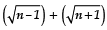is rational.
Hint:
If possible, let ‘n’ be a positive integer such that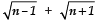is rational.

Let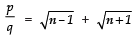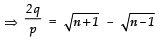(using componendo and dividendo)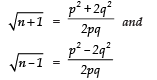Since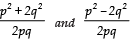are rational numbers.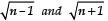are also rational.

i.e.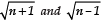are perfect squares of +ve integers. It is not possible.

[∵ two perfect squares differ by 3] ⇒  Our assumption is not possible.

Ques 3: If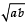is an irrational number, then show that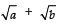is also irrational.
Hint:
If possible, let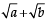be a rational number.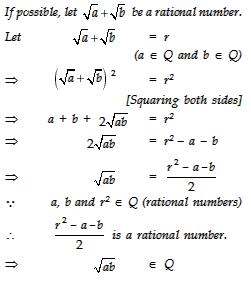But it contradicts our assumption that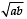is irrational.Thus,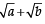is irrational.

Ques 4: How many prime numbers are there of the form 10n + 3, where n is a whole number 1 < n < 10?
Hint:
Substituting 1, 2, 3, .................... 10 in 10 n + 3, we have: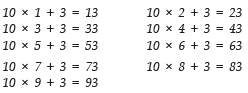Thus, the required numbers are: 13, 23, 43, 53, 73 and 83.

Offer running on EduRev: Apply code STAYHOME200 to get INR 200 off on our premium plan EduRev Infinity!

132 docs

,

,

,

,

,

,

,

,

,

,

,

,

,

,

,

,

,

,

,

,

,

;